Courses

# Floods - 2

## 10 Questions MCQ Test Topicwise Question Bank for GATE Civil Engineering | Floods - 2

Description
This mock test of Floods - 2 for Civil Engineering (CE) helps you for every Civil Engineering (CE) entrance exam. This contains 10 Multiple Choice Questions for Civil Engineering (CE) Floods - 2 (mcq) to study with solutions a complete question bank. The solved questions answers in this Floods - 2 quiz give you a good mix of easy questions and tough questions. Civil Engineering (CE) students definitely take this Floods - 2 exercise for a better result in the exam. You can find other Floods - 2 extra questions, long questions & short questions for Civil Engineering (CE) on EduRev as well by searching above.
QUESTION: 1

### The term ‘mean annual flood’ denote

Solution:

Gumbel’s distribution has the property which gives T = 2,33 years for the average of the annual series when N is very large. Thus the value of a flood with T = 2.33 years is called the mean annual flood.

QUESTION: 2

### Kirpich equation is used to determine which one, of the following?

Solution:

Kirpich equation is an empirical relation used for the estimation of the time of concentration. It is given as,
tc = 0.01947 L0.77 S-0.385
where, tc = time of concentration in minutes
L = maximum length of travel of water (m)
S = slope of the catchment = ΔH/L
ΔH = difference in elevation between the most remote point on the catchment outlet.

QUESTION: 3

### The confidence interval for the estimate of a value XT by Gumbel’s method depends on

Solution:
QUESTION: 4

A bridge has an expected life of 50 years and is designed for a flood magnitude of return period 100 years. What is the risk associated with this hydrologic design?

Solution:

Risk = Probability of flood being exceeded at least once over a period of n years.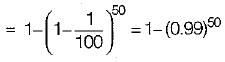QUESTION: 5

The use of the unit hydrograph for estimating floods is limited to catchments of size less than

Solution:
QUESTION: 6

Probability of a 10 year flood to occur at least once in the next 4 years is

Solution:

Probability corresponding to 10 year return period is,
P = 1/10 = 0.1
The probability of flood occurring at least once in 4 years is,
= 1 - (1 - P)4 = 1 - 0.94 = 0.3439 ≈ 35%

QUESTION: 7

The probable maximum flood is

Solution:

The Probable Maximum Precipitation (PMP) may be defined as the maximum depth of precipitation for a given duration that may possibly occur on a given catchment at any time of the year. Hence such a precipitation would result from the possible severest storm that may result from the worst possible combinations of hydrological conditions in the area.
Probable Maximum Flood (PMF) is the extreme flood that is physically possible in a region as a result of severe most combinations, including rare combinations of meteorological and hydrological factors. The PMF is used in situations where the failure of the structure would result in loss of life and catastrophic damage and as such complete security from potential floods is sought.

QUESTION: 8

The use of rational formula for estimating floods is limited to catchments of size less than

Solution:
QUESTION: 9

A hydraulic structure has been designed for a 50 year flood. The probability that exactly one flood of the design capacity will occur in the 75 year life of the structure is

Solution: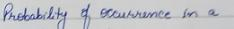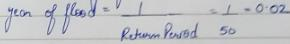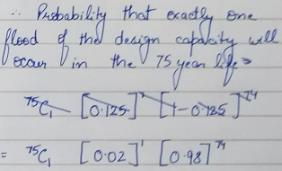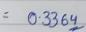QUESTION: 10

The standard project flood is

Solution:

The flood that would result from a sever combination of meteorological and hydrological factors that are reasonably applicable to the region. Extremely rare combinations of factors are excluded.